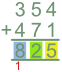# Adding In Columns

Basic, GCSE(F),

Adding increases the value of one number by the value of another.

Write one number underneath the other. Make sure that the digits are in columns, with each column representing a value: hundreds, tens, units.

Start by adding the numbers in the units column. Place the units figure of the answer at the bottom of the units column, and carry forward any tens figure to the next column.

Add the numbers in the tens column, including any carried forward number from the units addition. Place the tens figure of the answer at the bottom of the tens column, and carry forward any hundreds amount to the hundreds column.

Repeat the process for the hundreds column. Repeat again, if necessary, for the thousands column.

The same process can be used to add more than two numbers at the same time.

## Examples

1. Add 354 and 471.Add the units: 4 + 1 = 5.  Put the answer in the units column (shown in yellow).
Add the tens: 5 + 7 = 12.  From the answer, put the 2 in the tens column (green) and place the 1 in the hundreds column below the answer line (carry 1).
Add the hundreds, including the 1 brought forward: 3 + 4 + carried 1 = 8 (turquoise).

2. The coffee shop sold 5345 cups of coffee on Saturday and 2056 cups of coffee on Sunday. How many cups of coffee did the shop sell over the weekend?